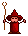###Author Topic: shortest distance between two lines (2D & 3D)  (Read 4052 times)

####r0ber7

• Prof. Inline
••• Posts: 540##### shortest distance between two lines (2D & 3D)
« on: 2013-Jul-12 »
While coding my physics engine, I found I needed an algorithm to calculate the closest distance between two lines. After some searching I found this, which I've converted into GLBasic.

This code will work in 2D and 3D. For 2D calculations, just enter zero for all z values.Code: GLBasic [Select]

TYPE point
x
y
z
ENDTYPE

TYPE geo_line
x1
y1
z1
x2
y2
z2
ENDTYPE

TYPE geometry

///*
//*
//* http://softsurfer.com/Archive/algorithm_0106/algorithm_0106.htm
//*
//* ===================
//* this code may be freely used AND modified FOR any purpose
//* providing that this copyright notice is included with it.
//* SoftSurfer makes no warranty FOR this code, AND cannot be held
//* liable FOR any real OR imagined damage resulting from its use.
//* Users of this code must verify correctness FOR their application.
//*/
FUNCTION norm : c1 AS point

LOCAL nrm = SQR(dot(c1, c1))
//console.out("geometry.norm> "+nrm+"\n")
RETURN nrm

ENDFUNCTION

FUNCTION dot : c1 AS point, c2 AS point

LOCAL dp = (c1.x * c2.x + c1.y * c2.y + c1.z * c2.z)
//console.out("geometry.dot> "+dp+"\n")
RETURN dp

ENDFUNCTION

FUNCTION linesegments_shortest_distance : line1 AS geo_line, line2 AS geo_line

LOCAL EPS = 0.00000001

LOCAL delta21 AS point
delta21.x = line1.x2 - line1.x1
delta21.y = line1.y2 - line1.y1
delta21.x = line1.z2 - line1.z1

LOCAL delta41 AS point
delta41.x = line2.x2 - line2.x1
delta41.y = line2.y2 - line2.y1
delta41.z = line2.z2 - line2.z1

LOCAL delta13 AS point
delta13.x = line1.x1 - line2.x1
delta13.y = line1.y1 - line2.y1
delta13.z = line1.z1 - line2.z1

LOCAL a = dot(delta21, delta21)
LOCAL b = dot(delta21, delta41)
LOCAL c = dot(delta41, delta41)
LOCAL d = dot(delta21, delta13)
LOCAL e = dot(delta41, delta13)
LOCAL D = a * c - b * b

LOCAL sc, sN, sD = D
LOCAL tc, tN, tD = D

IF D < EPS

sN = 0.0
sD = 1.0
tN = e
tD = c

ELSE

sN = (b * e - c * d)
tN = (a * e - b * d)
IF sN < 0.0

sN = 0.0
tN = e
tD = c

ELSEIF sN > sD

sN = sD
tN = e + b
tD = c
ENDIF
ENDIF

IF tN < 0.0

tN = 0.0

IF -d < 0.0
sN = 0.0
ELSEIF -d > a
sN = sD
ELSE

sN = -d
sD = a
ENDIF
ELSEIF tN > tD
tN = tD
IF (-d + b) < 0.0
sN = 0
ELSEIF (-d + b) > a
sN = sD
ELSE

sN = (-d + b)
sD = a
ENDIF
ENDIF

IF ABS(sN) < EPS
sc = 0.0
ELSE
sc = sN / sD
ENDIF

IF ABS(tN) < EPS
tc = 0.0
ELSE
tc = tN / tD
ENDIF

LOCAL dP AS point
dP.x = delta13.x + (sc * delta21.x) - (tc * delta41.x)
dP.y = delta13.y + (sc * delta21.y) - (tc * delta41.y)
dP.z = delta13.z + (sc * delta21.z) - (tc * delta41.z)

// shortest distance between two line segments

LOCAL sdist = SQR(dot(dP, dP))
//console.out("geometry.linesegments_shortest_distance> "+sdist+"\n")
RETURN sdist

ENDFUNCTION

« Last Edit: 2014-May-03 by Moru »

####bigsofty

• Community Developer
• Prof. Inline
••• Posts: 2640##### Re: shortest distance between two lines (2D & 3D)
« Reply #1 on: 2013-Jul-13 »
Many thanks!Cheers,

Ian.

“It is practically impossible to teach good programming style to students that have had prior exposure to BASIC.  As potential programmers, they are mentally mutilated beyond hope of regeneration.”
(E. W. Dijkstra)